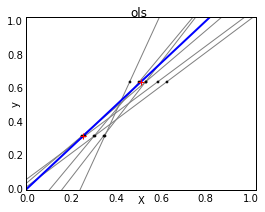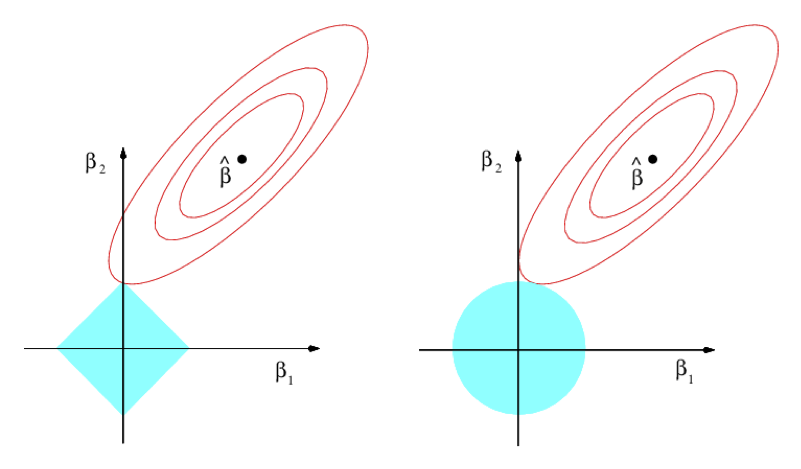Alex Egg,

# Occam’s Razor

Among competing hypotheses, the one with the fewest assumptions should be selected

## Question

What is a “complex” vs “simple” hypothesis?

A “simple” model is one where $\theta$ has few non-zero parameters. i.e.: only a few features are relevant

A “simple” model is one where $\theta$ is almost uniform. i.e: few features are significantly more relevant than the others

Regularization is the process of penalizing model complexity during training

# Regularization

Models w/ high bias will have a tendency to overfit the data or to learn the noise. One method to combat this is called regularization. You basically add a term to your cost function that penalizes large weights, which, in effect penalizes model complexity in training.

Equation 1 is your standard optimization problem that is often implemented as gradient descent. The First term is the standard residual measure that we are trying to minimize. The second term is the interesting part: the regularization. The coefficient $\lambda$ or sometimes $\alpha$ is simply a parameter we have to optimize. The interesting part is the norm of the weights vector: this is called the l2 Norm.

## L2 Norm

Consider the vectors $a=(0.5, 0.5)$ and $b=(-1,0)$. We can compute the L1 and L2 norms:

$\|a\|_1 = \|0.5\| + \|0.5\| = 1 \quad \textrm{and} \quad \|b\|_1 = |-1| + |0| = 1$
$\|a\|_2 = \sqrt{0.5^2 + 0.5^2} = 1/\sqrt{2} \quad \textrm{and} \quad \|b\|_2 = \sqrt{(-1^2 + 0^2} = 1$

As you can see, the two vectors are equivalent with respect to the L1 norm, however, the are different w/ respect to the L2 norm. This is because squaring the number punishes large values more than small values.

Often times the equation 1 is called “Tikhonov regularization” in academia or called Ridge in machine learning circles. For example, it is implemented as Ridge Regression in scikit. Ridge Regression really wants small value in all slots of $\theta$, whereas solving the L1 version doesn’t care if it’s large values or not.

## Analysis

So we have stated the L2 regularization helps to remove variance across the weights. Lets take a look at that in practice by comparing it to an unregularized linear regression.

# Code source: Gaël Varoquaux
# Modified by Alex Egg 12/15/16

import numpy as np
import matplotlib.pyplot as plt
%matplotlib inline

from sklearn import linear_model

X_train = np.c_[.5, 1].T
y_train = [.5, 1]
X_test = np.c_[0, 2].T

np.random.seed(0)

classifiers = dict(ols=linear_model.LinearRegression(),
ridge=linear_model.Ridge(alpha=.1))

fignum = 1
for name, clf in classifiers.items():
fig = plt.figure(fignum, figsize=(4, 3))
plt.clf()
plt.title(name)
ax = plt.axes([.12, .12, .8, .8])

for _ in range(6):
this_X = .1 * np.random.normal(size=(2, 1)) + X_train
clf.fit(this_X, y_train)

ax.plot(X_test, clf.predict(X_test), color='.5')
ax.scatter(this_X, y_train, s=3, c='.5', marker='o', zorder=10)

clf.fit(X_train, y_train)
ax.plot(X_test, clf.predict(X_test), linewidth=2, color='blue')
ax.scatter(X_train, y_train, s=30, c='r', marker='+', zorder=10)

ax.set_xticks(())
ax.set_yticks(())
ax.set_ylim((0, 1.6))
ax.set_xlabel('X')
ax.set_ylabel('y')
ax.set_xlim(0, 2)
fignum += 1

plt.show()Figure 1: Left is OLS regression sans regularization. You can see that the random variance added to the training data is exaggerated the regressions. Right is the OLS regression with ridge regularization. You can see that the random variance introduced into the training data has smaller effect not the regressions.

Source

“Due to the few points in each dimension and the straight line that linear regression uses to follow these points as well as it can, noise on the observations will cause great variance as shown in the first plot. Every line’s slope can vary quite a bit for each prediction due to the noise induced in the observations.

Ridge regression is basically minimizing a penalized version of the least-squared function. The penalizing shrinks the value of the regression coefficients. Despite the few data points in each dimension, the slope of the prediction is much more stable and the variance in the line itself is greatly reduced, in comparison to that of the standard linear regression.”

## Visualizing Regression

There is a compelling visualization and geometric argument for L1 and L2 regularization (ISLR Figure 6.7)Figure 2: On the left is Lasso and right is Ridge

There are 2 coefficients in this model where $\hat{\beta}$ is the least squares estimate on the 2 variables or the RSS minimum. As you move up the contours the RSS increases. The blue area is the constraint region which is a circle defined by the sum of squares. In ridge regression, you have a budget on the total sum of squares of the base. So the budget is the radius of a circle. Therefore the ridge problem says find the first place these contours hit the constraint region. In other words, find the smallest RSS you can get within the budget defined by this circle; this is where the sum of squares of $\beta_1$, $\beta_2$ is less than the budget. And since it is a circle you’d have to be very lucky to hit exactly the place where one or the other is 0.

Now, consider Lasso. Everything is same as the ridge except the constraint equation is now the sum of the absolute values. So rather than a circle, it’s a diamond. So in this picture, I’ve hit this corner, and now I get a place where $\hat{\beta_1}$ is 0.

So in other words, to summarize, the absolute value’s going to be a constraint region that has sharp corners. In high dimensions, you have edges and corners. And along an edge or a corner, if you hit there, you get a 0. So this is, geometrically, why you get sparsity in the Lasso.

## Takeaways

One measure of model complexity is the number of features. Another measure of model complexity is the size of the weights. Regularization is a method to control model complexity where Lasso and Ridge address those issues respectively.

If you are taking a regression using a linear method, depending on your data you should probably use a type of regularizer, either L1 (Lasso) or L2 (Ridge) to avoid a high number of low-variance features or to avoid features w/ high variance relative to the others, respectively.